Organic Chemistry : Using Other Organic Chemistry Lab Techniques

Example Questions

← Previous 1 3

Example Question #51 : Organic Chemistry

If the 1HNMR spectrum of the following compound is measured, how many signals will the spectrum feature?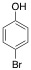Four

Six

Two

Three

Five

Three

Explanation:

In 1HNMR spectroscopy, each signal of a spectrum arises from a set of chemically unique hydrogens. Given the plane of symmetry through this molecule, only three sets of hydrogens are chemically unique. The figure below shows these three sets, where hydrogens of the same color are chemically equivalent, and will contribute to the same signal.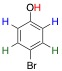Example Question #52 : Organic Chemistry

If the 1HNMR spectrum of the following compound is measured, what combination of various signal multiplicities will be featured?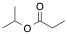A singlet, a doublet, a triplet, and a sextet

Two doublets, a triplet, and three quartets

A singlet, a doublet, and three triplets

A singlet, a doublet, a triplet, and two quartets

A doublet, a triplet, a quartet, and a septet

A doublet, a triplet, a quartet, and a septet

Explanation:

The molecule has four sets of chemically equivalent hydrogens, as shown in different colors in the diagram below, and thus will give rise to four unique signals.

The rule for determining the multiplicity (splitting) arising from a set of chemically identical hydrogens is "n +1," where "n" is the number of vicinal hydrogens. Remember that vicinal hydrogens are any hydrogens three bonds away from the hydrogen in question; these are the only hydrogens that are close enough to split the signal.

For example, the set of red hydrogens below have three vicinal hydrogens, shown in green. Count the "three bonds" that seperate these sets of hydrogens (red H to C, C to C, and C to green H) to verify you understand the concept of vicinal hydrogens. So, for the red hydrogens, n = 3, and because 3 + 1 = 4, they will give rise to a quartet signal.

Similarly, the green will give rise to a triplet, the orange will give rise to a doublet, and the blue will give rise to a septet.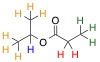Example Question #1 : Using Other Organic Chemistry Lab Techniques

A sample containing the product of an organic reaction is submitted for analysis via mass spectrometry. The results show a mass spectrum with a parent ion of 100 m/z units. Which of the following structures is a possible product compound?

1-fluoro-3,3-dimethylbutane

2-methylpent-3-en-1-amine

Hexan-3-one

4-methylpent-3-en-2-amine

Bromoethane

Hexan-3-one

Explanation:

This questions tests two skills. First, you must be able to convert from the names of compounds to either a drawing of the compound or a molecular formula. Secondly, you must then sum the atomic weights of all the atoms in the molecule to find a total molecular weight for each compound. The molecular weight of a compound is indicated in a mass spectrum of that compound as the m/z value of the parent peak, or peak with highest mass on the spectrum.

To find the correct answer, you must determine which of the answer choices give a molecule with a molecular weight of 100. Note, you may calculate the molecular weight to the nearest whole atomic mass unit (amu).

For example, the correct answer, hexan-3-one, shown below, has a chemical formula of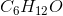. Because carbon weighs 12 amu, hydrogen weighs 1 amu, and oxygen weighs 16 amu, the molecular weight can be calculated as follows: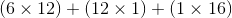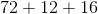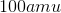The line diagram, chemical formula, and molecular mass to the nearest whole amu for all five answer choices are shown below.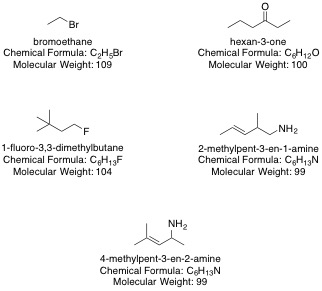Example Question #54 : Organic Chemistry

An organic chemist carries out a reaction she believes will install a carboxylic acid functional group in her substrate. To verify the reaction worked, she measures the infrared (IR) spectrum of the compound. Which of the following absorption features would confirm the presence of a carboxylic acid?

A weak band between 1700 and 1500 cm-1

A weak, single band below 675 cm-1

A broad, strong band between 3300 and 2500 cm-1

Several sharp, narrow bands between 3100 and 3000 cm-1

Several sharp, narrow bands between 3000 and 2850 cm-1

A broad, strong band between 3300 and 2500 cm-1

Explanation:

Infrared (IR) absorption spectra of carboxylic acids give very broad, strong bands between 3300 and 2500  cm-1 due to O-H bond stretching. This is similar to alcohols, which give a similar band.

The incorrect answers would indicate different types of functional groups, as detailed below:

Several sharp, narrow bands between 3100 and 3000 cm-1 indicate alkene functionality, and occur due to C-H bond stretching.

Several sharp, narrow bands between 3000 and 2850 cm-1 indicate alkane functionality, and occur due to C-H bond stretching.

A weak, single band below 675 cm is indicative of C-Br stretching.

A weak band between 1700 and 1500 cm-1 is indicative of aromatic C-C bond stretching.

Note: This answer choice is a red herring, because the energy range is similar to that of carbonyl C=O stretching, which should occur from 1780-1710 cm-1 for a carboxylic acid. However, as C=O absorptions are extremely sharp and strong, the descriptor "weak" makes this answer choice incorrect, in addition to the fact that the given range is slightly lower in energy than the typical carboxylic acid C=O stretch absorption.

Example Question #1 : Using Other Organic Chemistry Lab Techniques

An organic chemistry laboratory assistant was instructed to transfer 3.5mL of ethanol into an empty beaker. Which of the following pieces of equipment should the assistant use?

Buret

Pipet

Pipet

Explanation:

Pipets, when used correctly, are the most accurate pieces of equipment when transferring small quantities of liquid.

Example Question #56 : Organic Chemistry

What is the best instrument to use when transferring small amounts of acid into an unknown base during a titration?

Pipet

Buret

Buret

Explanation:

To perform a titration, one must use a buret to periodically release small amounts of a solution of known concentration into a solution of unknown concentration.

Example Question #51 : Organic Chemistry

An organic chemist begins to analyze an infrared (IR) spectrum and immediately notices a very strong peak at around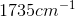. All of the following functional groups can correspond to this peak except __________.

Ether

Ester

Carboxylic acid

Amide

Ether

Explanation:

On an IR spectrum, a strong, sharp peak ranging from about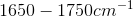indicates the presence of a carbonyl compound. Of the answer choices, ether is the only one that is not a carbonyl compound.

Example Question #2 : Using Other Organic Chemistry Lab Techniques

An organic chemist begins to analyze an ifrared (IR) spectrum. He immediately notices a strong, sharp peak at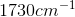. This peak is most likely present in the spectrum due to which of the following functional groups?

Amine

Alcohol

Aldehyde

Alkene

Aldehyde

Explanation:

This peak exists as a result of the presence of an aldehyde.

Any time that an IR spectrum shows a strong, sharp peak in between, we know that we have a carbonyl functional group present. The aldehyde is the only carbonyl out of the listed answer choices.

Example Question #59 : Organic Chemistry

An organic chemist begins to analyze an infrared (IR) spectrum. Immediately, he notices a strong, broad peak at around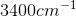. What functional group does peak belong to?

Alkyne

Alcohol

Carboxylic acid

Ketone

Alcohol

Explanation:

Any time that we see a strong, broad peak on an IR spectrum ranging from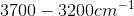, we know that an alcohol group is present in the compound that is being analyzed. Carbonyl compounds show strong, sharp peaks ranging from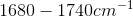. Alkynes show a weak, sharp peak from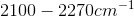.

Example Question #3 : Using Other Organic Chemistry Lab Techniques

An organic chemist began analyzing an infrared (IR) spectrum. She noticed a strong, sharp peak at around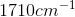and a strong, broad peak at around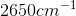. Which functional group(s) correspond(s) to the data provided by the IR spectrum?

Ketone and alcohol

Aldehyde and alcohol

Amide

Carboxylic acid

Carboxylic acid

Explanation:

The correct answer is carboxylic acid.

We know that the strong, sharp peak atmust correspond to a carbonyl group. This information does not help us much, however, as all of our answer choices contain at least one carbonyl.

The other defined peak atis the key to solving this problem. Strong, broad peaks are characteristic of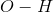bonds. However, this peak was absorbed at too low of a frequency to correspond to an alcohol group. Thus, our peaks must correspond to carboxylic acid, as it is the only answer choice that is a carbonyl group but that also contains anbond.

← Previous 1 3

All Organic Chemistry Resources